# Bonferroni inequalities

Jump to: navigation, search

A solution of the classical matching problem and the counting inclusion-and-exclusion method (cf. also Inclusion-and-exclusion principle) can be given in a unified manner by the following set of inequalities. Letbe events on a given probability space, and let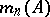denote the number of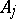that occur. Set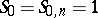and(a1)

where summation is over all subscripts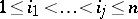. The numbers,, are known as the binomial moments of, since, by turning to indicators, one immediately obtains that, with the expectation operator,Now, the following inequalities are valid for all integersand:(a2)

Inequality (a2) can be rewritten forin the light of the elementary relation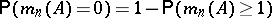. Furthermore, it can be extended to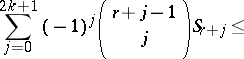(a3)and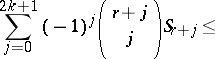(a4)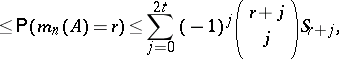whereis an arbitrary integer with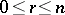and the limitsandsatisfy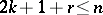and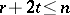.

Inequalities (a2), (a3) and (a4) are known as the Bonferroni inequalities because of their extensive use by C.E. Bonferroni [a1] in statistical settings; this work of Bonferroni generated a considerable follow-up in later years. However, the inequalities above were known earlier: for discrete probability spaces they go back to the eighteenth century, whilst for an abstract, and thus general, probability space their validity appears in [a3].

When the probability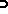is just counting proportions (a very typical case in number theory), then (a2) is known as the method of inclusion-and-exclusion. However, each of (a2), (a3) or (a4) is a very useful tool with a generally underlying probability in such widely ranging topics as combinatorics, number theory, information theory, statistics, and extreme value theory. For detailed descriptions of all such applications, see [a2].

Although the Bonferroni inequalities are very effective tools in several problems, they may become impractical in others. In particular, when the general terms of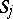are known with an error term, then, because of the large number of terms inas a function of, the error terms might dominate the sum of the main terms in the Bonferroni bounds, making the results meaningless. Modifications of the Bonferroni inequalities, known as Bonferroni-type inequalities, overcome this difficulty.